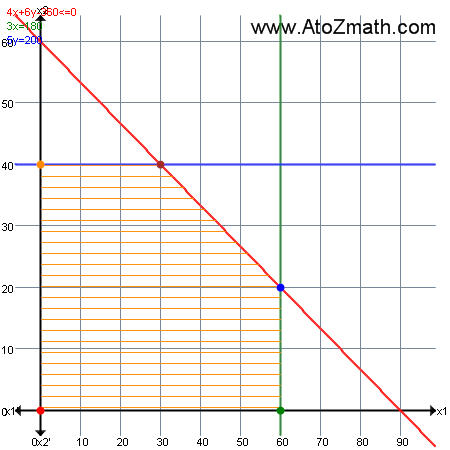Home > Operation Research calculators > Simplex method example

3. Graphical method example ( Enter your problem )

2. Example-2

Find solution using graphical method
MAX z = 15x1 + 10x2
subject to
4x1 + 6x2 <= 360
3x1 <= 180
5x2 <= 200
and x1,x2 >= 0

Solution:
Problem is
 MAX z_x =  15 x_1  +  10 x_2
subject to
  4 x_1  +  6 x_2 ≤ 360  3 x_1 ≤ 180  5 x_2 ≤ 200
and x_1,x_2 >= 0;

Hint to draw constraints

1. To draw constraint color{red}{4x_1+6x_2<=360 ->(1)}

Treat it as color{red}{4x_1+6x_2=360}

When x_1=0 then x_2=?

=>4(0)+6x_2=360

=>6x_2=360

=>x_2=(360)/(6)=60

When x_2=0 then x_1=?

=>4x_1+6(0)=360

=>4x_1=360

=>x_1=(360)/(4)=90

 x_1 0 90 x_2 60 0

2. To draw constraint color{green}{3x_1<=180 ->(2)}

Treat it as color{green}{3x_1=180}

=>x_1=(180)/(3)=60

Here line is parallel to Y-axis
 x_1 60 60 x_2 0 1

3. To draw constraint color{blue}{5x_2<=200 ->(3)}

Treat it as color{blue}{5x_2=200}

=>x_2=(200)/(5)=40

Here line is parallel to X-axis
 x_1 0 1 x_2 40 40The value of the objective function at each of these extreme points is as follows:
 Extreme PointCoordinates(x_1,x_2) Lines through Extreme Point Objective function valuez=15x_1 + 10x_2 color{red}{O(0,0)} color{black}{4->x_1>=0}color{black}{5->x_2>=0} 15(0)+10(0)=0 color{green}{A(60,0)} color{green}{2->3x_1<=180}color{black}{5->x_2>=0} 15(60)+10(0)=900 color{blue}{B(60,20)} color{red}{1->4x_1+6x_2<=360}color{green}{2->3x_1<=180} 15(60)+10(20)=1100 color{brown}{C(30,40)} color{red}{1->4x_1+6x_2<=360}color{blue}{3->5x_2<=200} 15(30)+10(40)=850 color{darkorange}{D(0,40)} color{blue}{3->5x_2<=200}color{black}{4->x_1>=0} 15(0)+10(40)=400

The maximum value of the objective function z=1100 occurs at the extreme point (60,20).

Hence, the optimal solution to the given LP problem is : x_1=60, x_2=20 and max z=1100.

This material is intended as a summary. Use your textbook for detail explanation.
Any bug, improvement, feedback then Submit Here